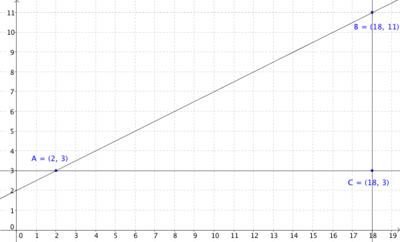## Alert!

Hello, reader! If you intend to post a link to this blog on Twitter, be aware that for utterly mysterious reasons, Twitter thinks this blog is spam, and will prevent you from linking to it. Here's a workaround: change the .com in the address to .ca. I call it the "Maple Leaf Loophole." And thanks for sharing!

## Saturday, August 16, 2014

### Zero and Undefined Slopes

Hey! I helped develop this task, which is intended to help students understand why horizontal lines have a slope of zero, and vertical lines have an undefined slope in service of CCSS standard 8.EE.B. It's based on Slopes between Points on a Line on Illustrative Mathematics. I'm publishing it here as a place to make it available. (If anyone reads this and thinks, "I know something way better that helps students achieve the same understanding," and it's easily accessible, please share it with me!)

The slope of the line joining two distinct points is calculated by finding the change in y-values and dividing by the change in x-values. For example, the slope between the points (7, -15) and (-8, 22) can be computed as follows:
• The difference in the y-values is −15 − 22 = −37.
• The difference in the x-values is 7 − (−8) = 15.
• Dividing these two differences, we find that the slope is 3715
The points A = (2, 3), B =(18,11) and C = (18,3) define three different lines: ABBC, and AC
1. One of the three lines has a slope of zero and one of the lines has an undefined slope. Determine which of the lines has slope zero and which has an undefined slope.
2. Create two points, D and E (different from AB, and C), that define a line whose slope is zero. State their coordinates and graph them on the coordinate grid along with line DE.
3. Create two points, F and G (different from the preceding points), so that the line joining F and G has undefined slope. State their coordinates and graph them along with line FG on the coordinate grid.
4. Describe the characteristics, in terms of coordinates and graphs, of lines that have a slope of zero.
5. Describe the characteristics, in terms of coordinates and graphs, of lines that will always have an undefined slope.

## Commentary

The "change in y divided by the change in x" can be computed for any two points in the plane... unless the points have the same x-coordinate. The purpose of this task is to help students understand why the calculated slope will be zero for any horizontal line and undefined for any vertical line.
This task is based on Slopes Between Points on a Line. In that task, students argue that for any two points on a line, the "slope triangles" have to be similar, and as a result the lengths of the sides of the triangles will be proportional. This is why the slope between any two points on a particular line will always be equal, and why we talk about "the" slope of a line. This task investigates a degenerate case where "slope triangles" can not be constructed, since the line is parallel to one of the axes.
This instructional task is intended to be used in a class discussion. Students can work on part (a) independently or in small groups. The class could discuss their answers before proceeding with the remaining parts. Students should be given a chance to try to create descriptions for part (d) and (e) on their own. Some may notice the graphical requirements, and some may notice the requirements of the coordinates. Both of these should be discussed and understood by the whole class, and the ideas should be connected to the slope-calculating procedure introduced at the beginning of the task.

## Solutiona. Possible response: Calculating the slope for AC, we get 33182=016=0. The slope of AC is 0. Calculating the slope of BC, we get 1131818=80, which is an undefined value. The slope of BC is undefined.

Another possible response: Looking at the graph of AB, I see that when x increases by 2, y increases by 1. I know that this means the slope of AB is 12. Looking at the graph of AC, I see that for every change of 1 unit in x, there is zero change in y. I suppose that this means the slope of AC is zero. When I look at the graph of BC, for every 1 unit of change in x, well, it's kind of crazy. All of the line happens when x is 18. I don't know how to say how y changes for every 1 x. I suppose this means the slope of BC is undefined.

b. Sample response:c. Sample Responsed. Lines whose slope is zero are horizontal. Two points on the line must have the same y-coordinate.

e. Lines with an undefined slope are vertical. Two points on the line must have the same x-coordinate.

#### Post a Comment

Hi! I will have to approve this before it shows up. Cuz yo those spammers are crafty like ice is cold.